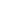This page has now been moved to Experiments with Colour and Brightnesses.
To Decide by Formula Whether Text Is Readable
This block is in black text on a mid-grey background, you’ll notice that it is not so clear to read as the white text on the same colour background in the next block, with the same font and size.
This block is in white text on a mid-grey background, it is surely noticeably clearer to read than the black text on the same colour background in the previous block, with same font and size.

The white text on mid-grey (RGB 128,128,128) is more clear to read than black text on mid-grey ( RGB 1,1,1 black so that the white and black are equidistant from grey 128, i.e. minus 127 and plus 127).
Why so? Shouldn’t the readability be the same, if the hues are the same and their brightnesses equidistant?
They would if the brightness of pixels on a screen increased linearly, but they do not increase linearly, there is a greater difference between two brightness levels on a bright pixel pair than a darker pair, a formula that is linear will never, never ever, give you a consistent result across the whole range of brightnesses.
This phenomenon, of relative brightness of bright pixels being perceptually greater than relative brightness of darker pixels, means that any formula that gives a brightness R, where R = G = B (i.e. red = green = blue colour values are equal and so the colour is grey) is not going to be useful when calculating the distance between two brightnesses, since the distance between the two brightnesses of given values will always be constant, irrespective of how bright or dark those relative values are. As my two panels above illustrate, this will not be a good determinant of readability.
ALL those formulas therefore which are of the type R*a + G*b + B*c, where a, b and c are each a constant, will be of limited use in determining readability of text over a background, because they do not take into account the different distance in relative brightness between darker and brighter pixels.
There are the gamma calculations, which work on an exponent and are described on my page Formula for Calculating Colour Brightness. The gamma calculation that gives a result identical to what you get with Photoshop still gives a brightness R where R = G = B. Although the formula uses exponents, the brightness results come out linear, see my page Colour Brightness Experiment. This is obvious when you consider the 256 shades of grey, any one of which gets a brightness value equal to its brightness number, i.e. a grey of value 80, say, will have a brightness value of 80. As the two panels above show, this is inadequate for determining relative brightness perception.
In order to gauge relative brightness, the brightnesses need to be weighted, to give a lower value the darker the brightness value. This is demonstrated on my page Colour Brightness Experiment and on my page Text Readability in Colour. For example, unweighted, the following two panels both have the same brightness difference.
This block is in green text on a red background, the background brightness values are 132 in like-Photoshop gamma, 98 in linear CIE XYZ, and 113 in linear YIQ. Text brightness values 192 like-Photoshop gamma (diff 60) 174 in linear CIE XYZ (diff 76) and 153 in linear YIQ (diff 40).
This block is in red text on a green background, the background brightness values are 132 in like-Photoshop gamma, 123 in linear CIE XYZ, and 111 in linear YIQ. Text brightness values 192 like-Photoshop gamma (diff 60) 187 in linear CIE XYZ (diff 64) and 193 in linear YIQ (diff 82).

The brightness differences are the same (in the like-Photoshop gamma formula) but their perceptual difference clearly isn’t. Most people will, I think, find the rightmost panel much more comfortable to read than the leftmost.
Now we do the same backgrounds, but use a weighted formula with an exponent of 2.5 (see my page Colour Brightness Experiment. The brightness difference in the like-Photoshop formula is, as above, 60 in both cases, though this time it has been weighted.
This block is in green text on a red background, the background brightness values are 49 in like-Photoshop gamma, 23 in linear CIE XYZ, and 34 in linear YIQ. Text brightness values 109 like-Photoshop gamma (diff 60) 81 in linear CIE XYZ (diff 59) and 67 in linear YIQ (diff 34).
This block is in red text on a green background, the background brightness values are 49 in like-Photoshop gamma, 41 in linear CIE XYZ, and 32 in linear YIQ. Text brightness values 109 like-Photoshop gamma (diff 60) 100 in linear CIE XYZ (diff 49) and 111 in linear YIQ (diff 79).

Is that better? Yes I think it looks much more consistent. And green on red of similar brightness levels is an extreme example to demonstrate.
And how about greys? Weighted with an exponent of 2.5, mid-grey comes out at R=G=B=194.
This block is in black text (R=G=B=42) on a grey background, the background brightness values are 129 in all calculation formulas (you cannot get 128). Text brightness values 3 in all formulas (diff 126).
This block is in white text on a grey background, the background brightness values are 129 all formulas. Text brightness values are 255 in all formulas (diff 126). The brightness difference is therefore the same in both panels.

That’s more like it.
The menu down the right of the page points to various experiments with colour brightness calculations, being the part-completed fruits of my researches. See also Text Readability in Colour.